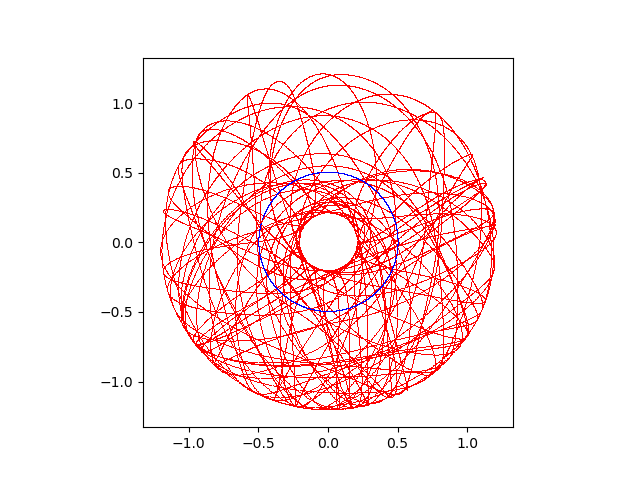## 使用Open Dynamics Engine实现刚体动力学仿真

#### 1. ODE的安装

ODE的安装非常简单，从其官方网站https://www.ode.org，找到在BitBucket中的代码库地址，使用git直接下载整个代码库，并使用cmake进行编译安装。此外也可以使用各Linux发行版的包管理器进行安装。

pacman -S ode
#下载源代码
git clone https://bitbucket.org/odedevs/ode.git
cmake .
make


python setup.py build
python setup.py install


#### 2. ODE的模块组成

ODE中对于整个模型分为世界World，刚体Body，约束（铰接）Joints ，力Force，几何体Geometry等。通过在World中增加Boby及Joints等连接约束关系，建立整个多刚体等力学模型，并通过不断循环时间步长通过积分来计算整个系统的下个状态。代码的基本框架如下

import ode;
#创建时间
world = ode.World();
#设置重力
world.setGravity((0, -9.8, 0));
#新建一个刚体
b1 = ode.Body(world);
#设置时间补偿
TIME_STEP = 0.01;
#进行1000次积分循环
for i in range(0, 1000):
#得到当前刚体的位置
x1, y1, z1 = b1.getPosition();
world.step(TIME_STEP);


#### 3. 双摆的运动模拟及双摆曲线的绘制

import numpy;
import ode;
from matplotlib import pyplot as plt;
import matplotlib.animation as animation;
if __name__ == '__main__':
#创建世界
world = ode.World();
world.setGravity((0, -9.8, 0));
#创建第一个质点
b1 = ode.Body(world);
#设置初始位置和速度
b1.setPosition((0, -0.5, 0))
b1.setLinearVel((10, 0, 0));
#设置质量和外形
m1 = ode.Mass();
m1.setSphereTotal(0.1, 0.05);
b1.setMass(m1);
#创建第二个质点
b2 = ode.Body(world);
#设置初始位置，默认初始速度为0
b2.setPosition((0.5, -1.0, 0));
#设置质量和外形
m2 = ode.Mass();
m2.setSphereTotal(0.1, 0.05);
b2.setMass(m2);
#创建第一个质点和环境的约束
j1 = ode.BallJoint(world);
j1.attach(b1, ode.environment);
j1.setAnchor((0.0, 0.0, 0.0));
#创建第二个质点和第一个质点之间的约束
j2 = ode.BallJoint(world);
j2.attach(b1, b2);
j2.setAnchor((0, -0.5, 0));
#设置时间步长
TIME_STEP = 0.01;
fig, ax = plt.subplots();
for i in range(0, 20000):
#分别获得两个质点的位置
x1, y1, z1 = b1.getPosition();
x2, y2, z2 = b2.getPosition();
#绘制当前的位置
plt.plot(x1, y1, 'b,');
plt.plot(x2, y2, 'r,');
#进入下一时刻
world.step(TIME_STEP);
#设置绘图的X和Y轴保持相同的比例
ax.set_aspect('equal');
#保持绘制的图片
plt.savefig('1.png');